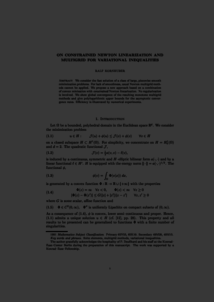Repository: Freie Universität Berlin, Math Department

# On constrained Newton linearization and multigrid for variational inequalities

Kornhuber, R. (2002) On constrained Newton linearization and multigrid for variational inequalities. Numerische Mathematik, 91 (4). pp. 699-721. ISSN 0029-599XPreview

1MB

Official URL: http://dx.doi.org/10.1007/s002110100341

## Abstract

We consider the fast solution of a class of large, piecewise smooth minimization problems. For lack of smoothness, usual Newton multigrid methods cannot be applied. We propose a new approach based on a combination of convex minization with constrained Newton linearization. No regularization is involved. We show global convergence of the resulting monotone multigrid methods and give polylogarithmic upper bounds for the asymptotic convergence rates. Efficiency is illustrated by numerical experiments.

Item Type: Article Mathematical and Computer Sciences > Mathematics > Numerical Analysis Department of Mathematics and Computer Science > Institute of Mathematics 1838 Ekaterina Engel 06 Mar 2016 12:34 03 Mar 2017 14:42

Repository Staff Only: item control page